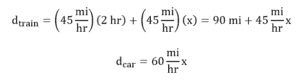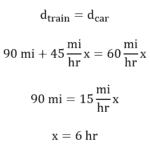# PCAT Quantitative Reasoning – Train and Car Speeds

• by
• Apr 19, 2018
• PCAT Question of the Day

A train travels at a speed of 45 mph in the west direction. Suppose a car leaves from the same origin two hours later, traveling 60 mph in the same direction. After how many hours from the car’s departure will the car catch up to the train?

A. 2
B. 4
C. 6
D. 8

##### Click for Explanation

C is correct. If both the train and the car leave from the same origin, they will have covered the same distance at the point that they meet, so we can set the distance the train travels equal to the distance the car travels. Let’s set equations for the distances the train and the car travel:Now, let’s set these equal to each other, solving for x, which is the number of hours the car travels to catch up with the train: